# Lions Weekly: September 7, 2022# A Note From Mr. Sandefur

Lion Family,

Good afternoon! I hope you and your family had a restful long week. I am writing to you today from our second annual college road trip for our juniors and seniors. A group of twenty-four students and three faculty members are visiting six colleges and universities. We are visiting Johnson University, Appalachian State University (Boone, NC), Wake Forest University (Winston-Salem, NC), University of North Carolina (Chapel Hill, NC), Duke University (Durham, NC), Liberty University (Lynchburg, VA), as we seek to expose students to potential opportunities for the next steps in their educational journey. This group has represented TKA exceptionally well and is looking forward to the remainder of the visits on this trip.

I’d ask that you take some time this week to specifically pray for our juniors and seniors as they seek the Lord’s will for the next steps. We are joining their families in prayer for wisdom, provision, and discernment.

In Christ Alone,

Jeremy Sandefur

 table div table+table+table+table div table{width:100%;padding:0}table div table+table+table+table div table img{width:96.23%;padding:0;float:none}table div table+table+table+table div table td{width:100%;padding:0 1.88% 18px}/* styles */# Mum Pick Up

## Tomorrow, September 8

Mums will be available for pick up tomorrow, Thursday, September 8 from 2PM-7PM at the soccer field by Ogle Building.

Thank you to everyone who supported our PTF by purchasing a mum!

# Mum Top Sellers

Congratulations to our two top sellers of Mums, Ruthie Smith and Mason Pugh!

Ruthie sold 127 mums and will be the crown holder at the Homecoming game.

Mason sold 83 mums and will be the ball holder at the Homecoming game.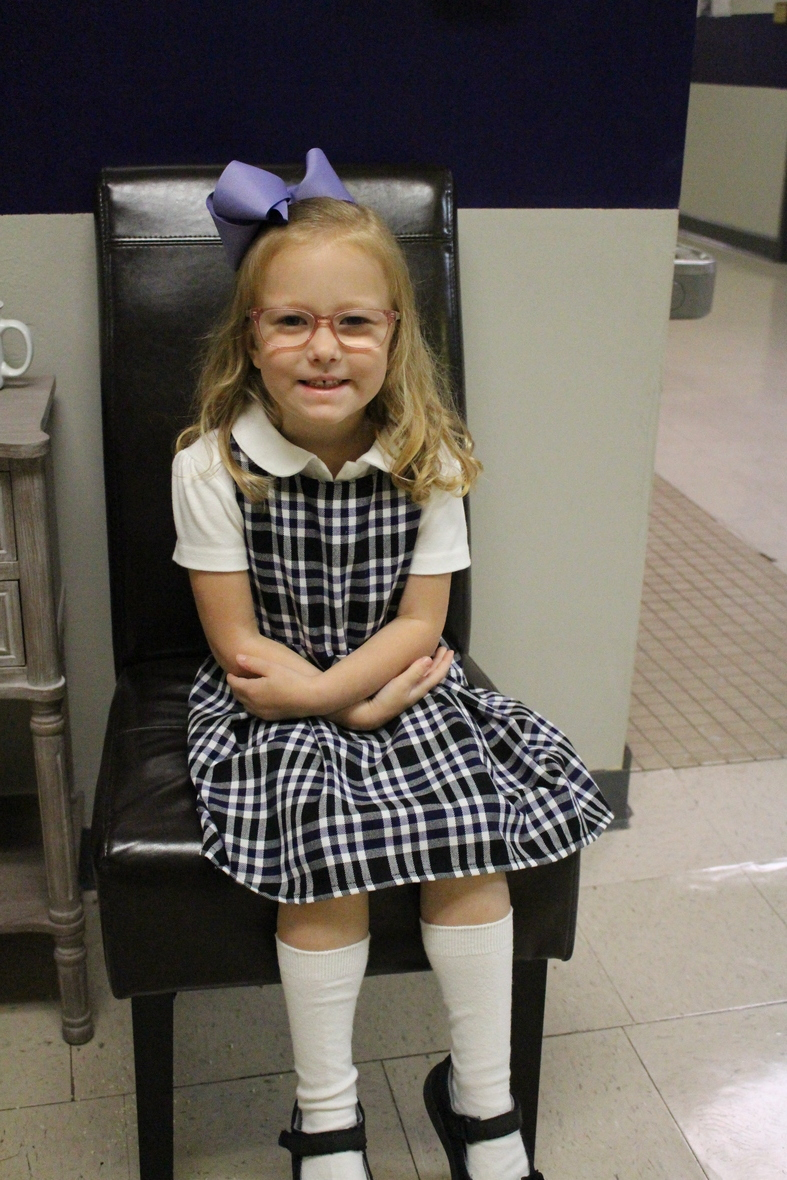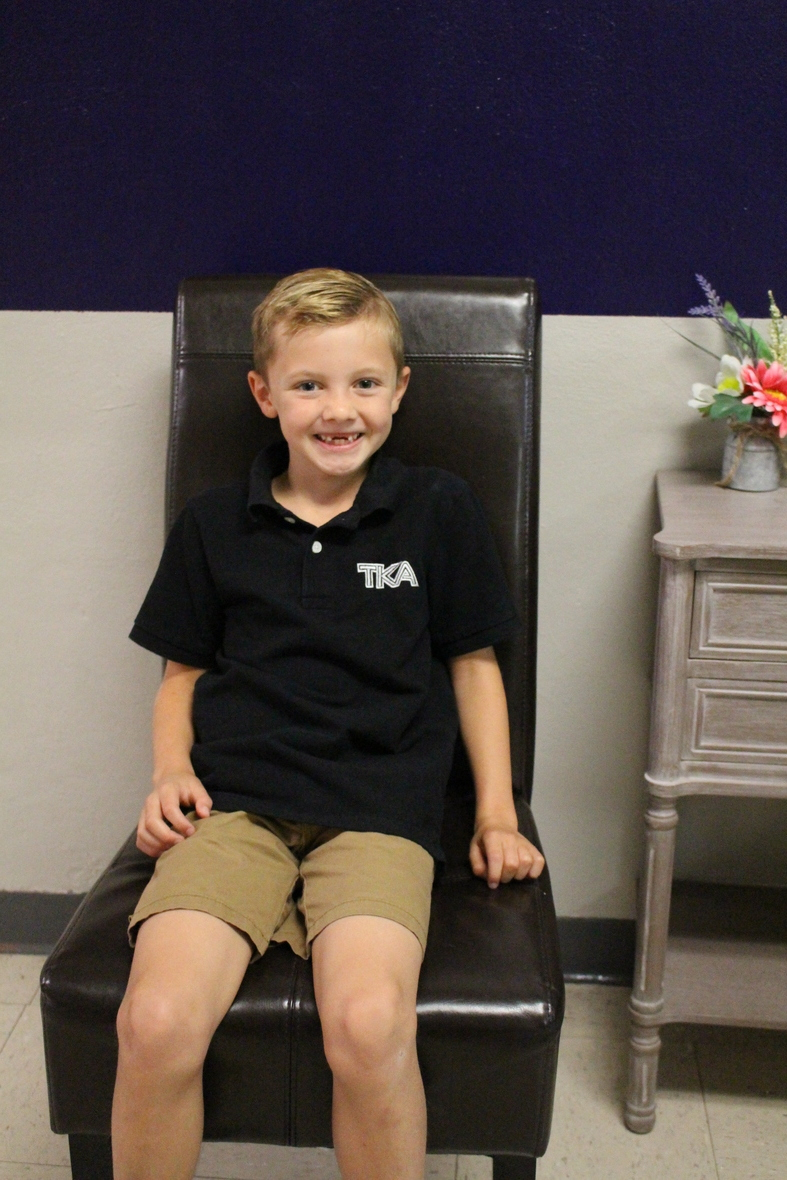table div table+table+table+table+table+table+table+table div table{width:100%;padding:0}table div table+table+table+table+table+table+table+table div table img{width:96.23%;padding:0;float:none}table div table+table+table+table+table+table+table+table div table td{width:100%;padding:0 1.88% 18px}/* styles */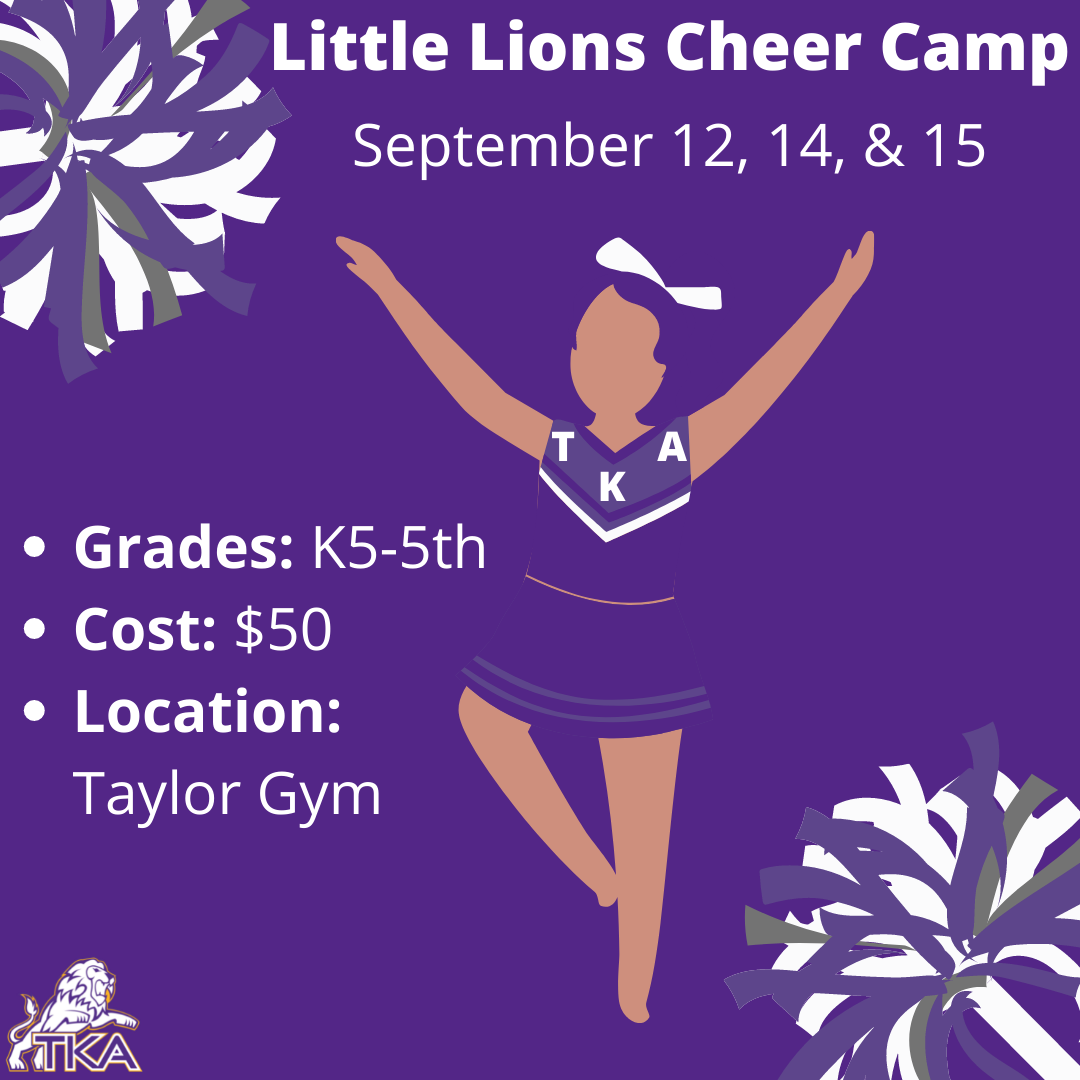# Little Lion Cheer Camp

## September 12, 14, & 15

▪ When: September 12, 14, &15
▪ Time: 3:15 p.m. to 4:30 p.m.
▪ Cost: \$50 (includes snacks and a t-shirt)
▪ Little Lions who attend camp will perform on Friday, September 16, at the home game against Friendship Christian.
 ▪ When: September 12, 14, &15
 ▪ Time: 3:15 p.m. to 4:30 p.m.
 ▪ Cost: \$50 (includes snacks and a t-shirt)
 ▪ Little Lions who attend camp will perform on Friday, September 16, at the home game against Friendship Christian.
 table div table+table+table+table+table+table+table+table+table+table+table div table{width:100%;padding:0}table div table+table+table+table+table+table+table+table+table+table+table div table img{width:96.23%;padding:0;float:none}table div table+table+table+table+table+table+table+table+table+table+table div table td{width:100%;padding:0 1.88% 18px}/* styles */Is your 2nd - 5th grader interested in playing basketball for TKA's Jr. League teams this year? Please complete this brief 'TKA Jr. League Basketball Pre-Registration Form' that will assist us in determining interest and developing preliminary rosters. Once completed, our Jr. League leadership team will evaluate the responses and reach out to you with additional information.

Jr. League is a competitive elementary basketball league which typically consists of 1-2 practices per week. Games begin in early November in gyms throughout Sevier County (TKA included) and involve games or tournaments each weekend through mid-February.

We look forward to hearing from you!

tkahoops@gmail.com

 table div table+table+table+table+table+table+table+table+table+table+table+table+table+table div table{width:100%;padding:0}table div table+table+table+table+table+table+table+table+table+table+table+table+table+table div table img{width:96.23%;padding:0;float:none}table div table+table+table+table+table+table+table+table+table+table+table+table+table+table div table td{width:100%;padding:0 1.88% 18px}/* styles */# Elementary Clubs

Elementary Clubs will start next week! Club teachers will email families by this Friday with a start date for each club.

 table div table+table+table+table+table+table+table+table+table+table+table+table+table+table+table+table div table{width:100%;padding:0}table div table+table+table+table+table+table+table+table+table+table+table+table+table+table+table+table div table img{width:96.23%;padding:0;float:none}table div table+table+table+table+table+table+table+table+table+table+table+table+table+table+table+table div table td{width:100%;padding:0 1.88% 18px}/* styles */# Jr. Beta Bake Sale

## September 12 - 16

Each day next week, our Jr. Beta members will be set up at the Reagan Pavilion from 10:30 to 12:30 selling baked goods.

Everything will cost \$1 and proceeds will go towards taking Jr Beta Club to represent TKA at TN State Convention in November.

 table div table+table+table+table+table+table+table+table+table+table+table+table+table+table+table+table+table+table div table{width:100%;padding:0}table div table+table+table+table+table+table+table+table+table+table+table+table+table+table+table+table+table+table div table img{width:96.23%;padding:0;float:none}table div table+table+table+table+table+table+table+table+table+table+table+table+table+table+table+table+table+table div table td{width:100%;padding:0 1.88% 18px}/* styles */# Grandparents' Day 2022

We are extremely excited to share with you that Grandparents’ Day is returning to TKA this fall on Friday, September 23, 2022. We understand the importance of sharing these integral moments between child and grandparents to create lasting memories. We are thrilled to welcome back all grandparents to TKA for this special event.

Grandparents are invited to arrive with their grandchild(ren) at 7:45 a.m. to check in with their little lion’s teacher in the classroom to enjoy coffee, refreshments, fellowship, and an activity. After time in the classroom, grandparents are invited to attend our Grandparents’ Day Program hosted in TKA Tibbetts Gymnasium. We ask for our grandparents to arrive in Tibbetts by 8:40 a.m. to receive a token of our appreciation for your dedication and support for TKA. Any middle/high school sibling may also attend the program with their grandparents. These students must be given permission on the form below to be dismissed from class. The Grandparents’ Day Program will include our elementary student body leading in worship, our HS Chapel Band, a song by our MS/HS Choir, followed by a word of encouragement from a Guest Speaker. Following the program, students who are given parent permission via the Student Release Form, will be allowed to dismiss with their grandparents. Grandparents are encouraged to visit our Book Fair and tour the campus before they leave. These opportunities will be available until 12:00 p.m. to allow our remaining students to continue their normal academic schedule.

Please RSVP on the link below by Friday, September 16th. This link will also be used for parent permission for student release. The student release will allow a student’s grandparent to check them out of school following the program. On the day of the event, grandparents will sign out your child in the classroom with your child’s teacher, not the elementary office.

 /* styles */ If you have any questions, please contact me at ksullivan@thekingsacademy.net. We are looking forward to a wonderful time of fellowship with all grandparents. Blessings, Kelly Sullivan, Elementary Principal
 table div table+table+table+table+table+table+table+table+table+table+table+table+table+table+table+table+table+table+table+table+table+table div table{width:100%;padding:0}table div table+table+table+table+table+table+table+table+table+table+table+table+table+table+table+table+table+table+table+table+table+table div table img{width:96.23%;padding:0;float:none}table div table+table+table+table+table+table+table+table+table+table+table+table+table+table+table+table+table+table+table+table+table+table div table td{width:100%;padding:0 1.88% 18px}/* styles */# PTF Coffee Mingle

PTF would like to invite members and those who would like to join PTF for coffee and conversation. So, mark your calendars and save the date for the PTF Coffee Mingle on Friday, September 23, at The Bridge Coffee House.

 table div table+table+table+table+table+table+table+table+table+table+table+table+table+table+table+table+table+table+table+table+table+table+table+table div table{width:100%;padding:0}table div table+table+table+table+table+table+table+table+table+table+table+table+table+table+table+table+table+table+table+table+table+table+table+table div table img{width:96.23%;padding:0;float:none}table div table+table+table+table+table+table+table+table+table+table+table+table+table+table+table+table+table+table+table+table+table+table+table+table div table td{width:100%;padding:0 1.88% 18px}/* styles */table div table+table+table+table+table+table+table+table+table+table+table+table+table+table+table+table+table+table+table+table+table+table+table+table+table+table div table{width:100%;padding:0}table div table+table+table+table+table+table+table+table+table+table+table+table+table+table+table+table+table+table+table+table+table+table+table+table+table+table div table img{width:96.23%;padding:0;float:none}table div table+table+table+table+table+table+table+table+table+table+table+table+table+table+table+table+table+table+table+table+table+table+table+table+table+table div table td{width:100%;padding:0 1.88% 18px}/* styles */# Fall 2022 Dress Up Day Themes

Remember, all attire must adhere to dress code

▪ Friday, September 16 - Hunting for a Repeat (Dress in Camo)
▪ Thursday, September 22 - Gold Out (Dress in Gold for Childhood Cancer Awareness)
▪ Thursday, October 6 - Pink Out (Dress in Pink for Breast Cancer Awareness)
▪ Tuesday, October 18 - Friday, October 21 - Homecoming Week (see details below)
▪ Friday, October 28 - "Senior" Spirit Day (Dress in "Senior" clothes in honor of Senior night)
 ▪ Friday, September 16 - Hunting for a Repeat (Dress in Camo)
 ▪ Thursday, September 22 - Gold Out (Dress in Gold for Childhood Cancer Awareness)
 ▪ Thursday, October 6 - Pink Out (Dress in Pink for Breast Cancer Awareness)
 ▪ Tuesday, October 18 - Friday, October 21 - Homecoming Week (see details below)
 ▪ Friday, October 28 - "Senior" Spirit Day (Dress in "Senior" clothes in honor of Senior night)

## Homecoming Week: Blast from the Past

▪ Tuesday, October 18 - 90s Dress Up Day (Dress in 90s attire from movies, TV shows, musicians, or sports stars)
▪ Wednesday, October 19 - 80s Dress Up Day (Dress in 80s attire from movies, TV shows, musicians, or sports stars)
▪ Thursday, October 20 - 70s Dress Up Day (Dress in 70s attire from movies, TV shows, musicians, or sports stars)
▪ Friday, October 21 - Homecoming T-shirt or TKA Spirit attire
 ▪ Tuesday, October 18 - 90s Dress Up Day (Dress in 90s attire from movies, TV shows, musicians, or sports stars)
 ▪ Wednesday, October 19 - 80s Dress Up Day (Dress in 80s attire from movies, TV shows, musicians, or sports stars)
 ▪ Thursday, October 20 - 70s Dress Up Day (Dress in 70s attire from movies, TV shows, musicians, or sports stars)
 ▪ Friday, October 21 - Homecoming T-shirt or TKA Spirit attire
 table div table+table+table+table+table+table+table+table+table+table+table+table+table+table+table+table+table+table+table+table+table+table+table+table+table+table+table+table+table div table{width:100%;padding:0}table div table+table+table+table+table+table+table+table+table+table+table+table+table+table+table+table+table+table+table+table+table+table+table+table+table+table+table+table+table div table img{width:96.23%;padding:0;float:none}table div table+table+table+table+table+table+table+table+table+table+table+table+table+table+table+table+table+table+table+table+table+table+table+table+table+table+table+table+table div table td{width:100%;padding:0 1.88% 18px}/* styles */# 2021-22 Yearbooks

Preordered yearbooks, are in and delivered to students. If you did not order a yearbook for 2021-22, they can be purchased for \$55 in either school office or by emailing Mr. Josh Smith jsmith@thekingsacademy.net.

 table div table+table+table+table+table+table+table+table+table+table+table+table+table+table+table+table+table+table+table+table+table+table+table+table+table+table+table+table+table+table+table div table{width:100%;padding:0}table div table+table+table+table+table+table+table+table+table+table+table+table+table+table+table+table+table+table+table+table+table+table+table+table+table+table+table+table+table+table+table div table img{width:96.23%;padding:0;float:none}table div table+table+table+table+table+table+table+table+table+table+table+table+table+table+table+table+table+table+table+table+table+table+table+table+table+table+table+table+table+table+table div table td{width:100%;padding:0 1.88% 18px}/* styles */# 9-12th Spring Break Trip: France, Germany and The Alps

## March 11-18, 2023

 table div table+table+table+table+table+table+table+table+table+table+table+table+table+table+table+table+table+table+table+table+table+table+table+table+table+table+table+table+table+table+table+table+table div table{width:100%;padding:0}table div table+table+table+table+table+table+table+table+table+table+table+table+table+table+table+table+table+table+table+table+table+table+table+table+table+table+table+table+table+table+table+table+table div table img{width:96.23%;padding:0;float:none}table div table+table+table+table+table+table+table+table+table+table+table+table+table+table+table+table+table+table+table+table+table+table+table+table+table+table+table+table+table+table+table+table+table div table td{width:100%;padding:0 1.88% 18px}/* styles */# Weekly Sports Schedule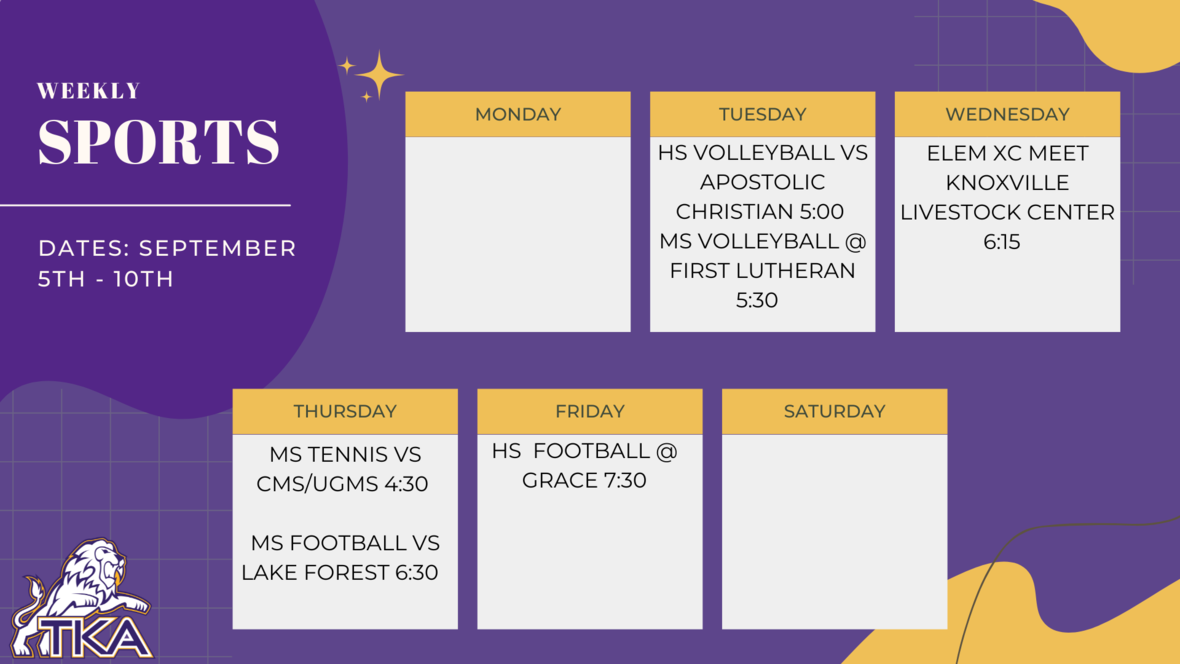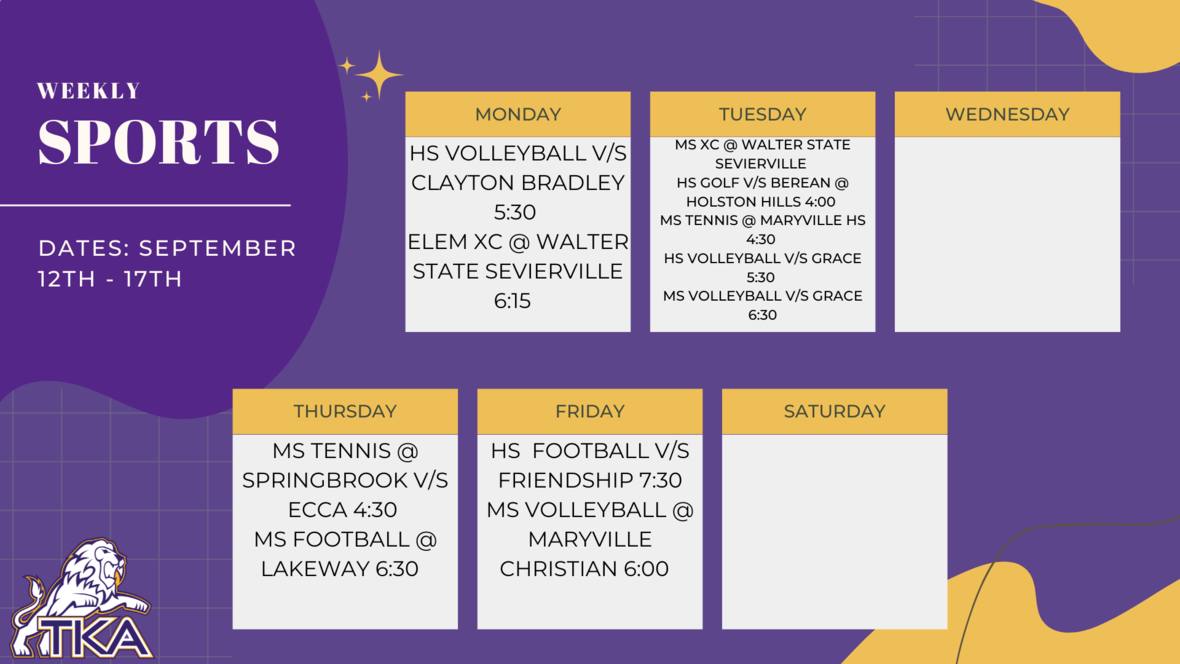table div table+table+table+table+table+table+table+table+table+table+table+table+table+table+table+table+table+table+table+table+table+table+table+table+table+table+table+table+table+table+table+table+table+table+table+table+table div table{width:100%;padding:0}table div table+table+table+table+table+table+table+table+table+table+table+table+table+table+table+table+table+table+table+table+table+table+table+table+table+table+table+table+table+table+table+table+table+table+table+table+table div table img{width:96.23%;padding:0;float:none}table div table+table+table+table+table+table+table+table+table+table+table+table+table+table+table+table+table+table+table+table+table+table+table+table+table+table+table+table+table+table+table+table+table+table+table+table+table div table td{width:100%;padding:0 1.88% 18px}/* styles */# Important information for the 2022-2023 School Year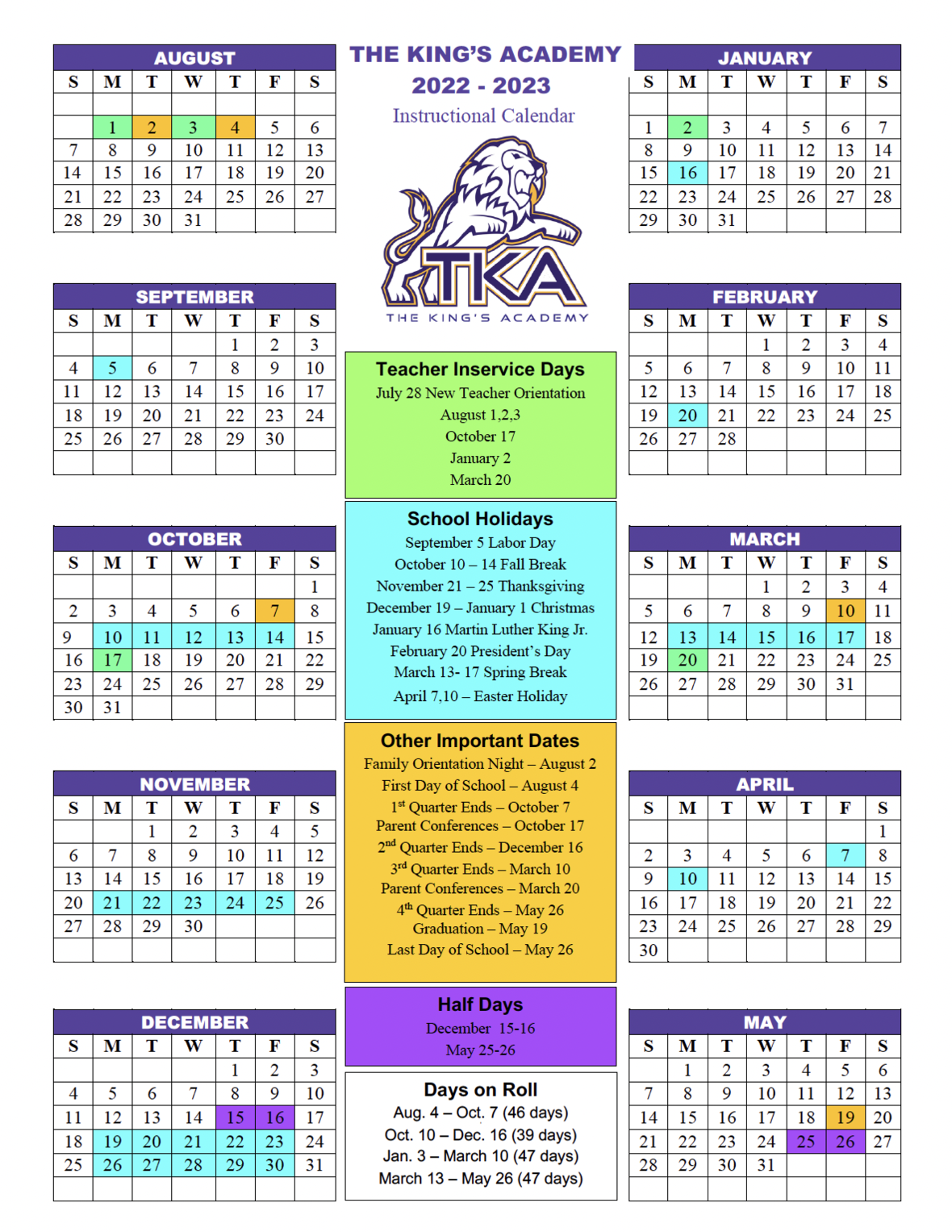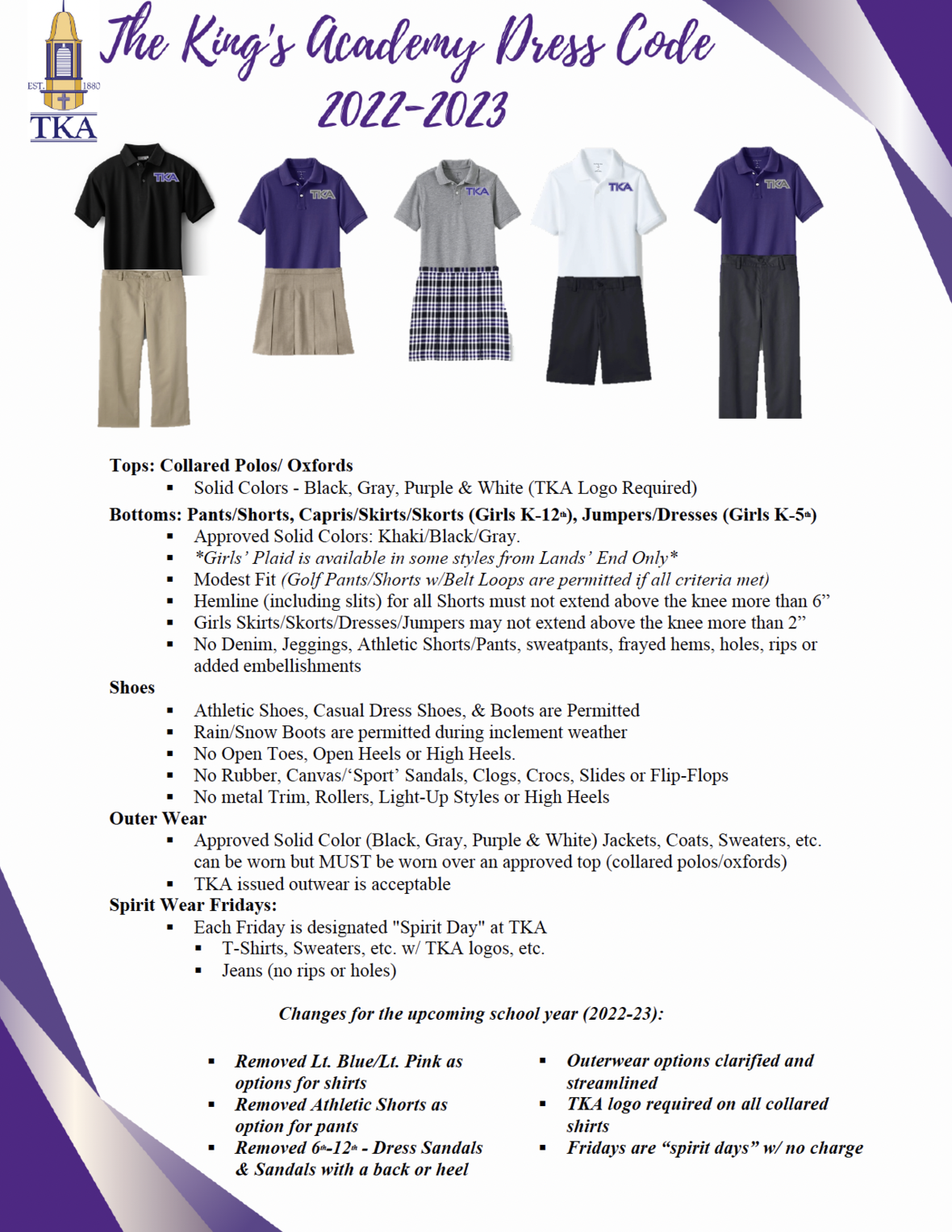table div table+table+table+table+table+table+table+table+table+table+table+table+table+table+table+table+table+table+table+table+table+table+table+table+table+table+table+table+table+table+table+table+table+table+table+table+table+table+table+table+table div table td,table.module-40{width:100%;padding:0}table div table+table+table+table+table+table+table+table+table+table+table+table+table+table+table+table+table+table+table+table+table+table+table+table+table+table+table+table+table+table+table+table+table+table+table+table+table+table+table+table+table div table{width:100%;float:none;margin-left:auto;margin-right:auto;padding:0}table div table+table+table+table+table+table+table+table+table+table+table+table+table+table+table+table+table+table+table+table+table+table+table+table+table+table+table+table+table+table+table+table+table+table+table+table+table+table+table+table+table div table a{border:0 none;text-decoration:none}table div table+table+table+table+table+table+table+table+table+table+table+table+table+table+table+table+table+table+table+table+table+table+table+table+table+table+table+table+table+table+table+table+table+table+table+table+table+table+table+table+table div table img{width:100%!important;border:0 none;text-decoration:none}/* styles */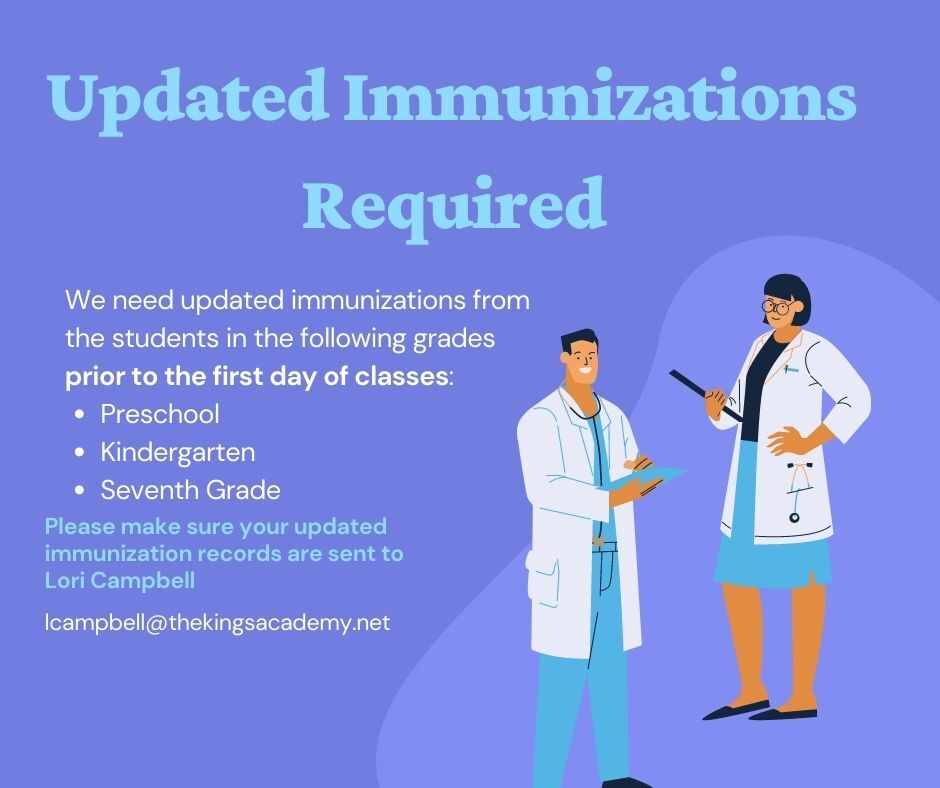table div table+table+table+table+table+table+table+table+table+table+table+table+table+table+table+table+table+table+table+table+table+table+table+table+table+table+table+table+table+table+table+table+table+table+table+table+table+table+table+table+table+table+table div table{width:100%;padding:0}table div table+table+table+table+table+table+table+table+table+table+table+table+table+table+table+table+table+table+table+table+table+table+table+table+table+table+table+table+table+table+table+table+table+table+table+table+table+table+table+table+table+table+table div table img{width:96.23%;padding:0;float:none}table div table+table+table+table+table+table+table+table+table+table+table+table+table+table+table+table+table+table+table+table+table+table+table+table+table+table+table+table+table+table+table+table+table+table+table+table+table+table+table+table+table+table+table div table td{width:100%;padding:0 1.88% 18px}/* styles */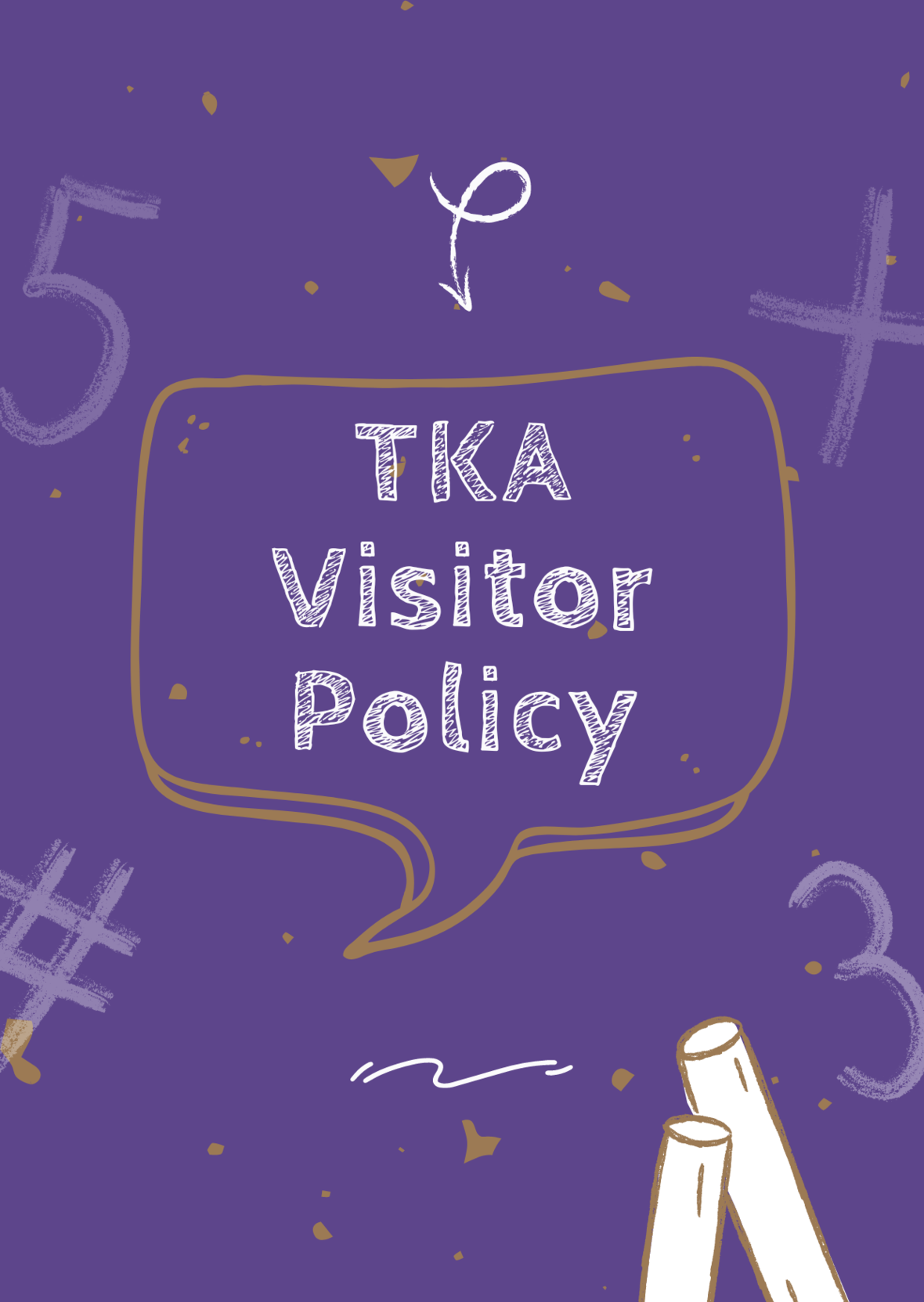# TKA Visitor Policy

In order to help maintain a safe and instructionally based learning environment for our students, all visitors for middle and high school must enter through the front door of the Anderson Building and sign in in the middle/high school office in order to receive a visitor's pass. All visitors for elementary must enter the front door of the Ogle Building in order to receive a visitor's pass. This pass should be visible at all times so that TKA faculty and staff know that you have checked in at the office.

Our doors are open to visitors, but please call ahead of time to schedule conferences with your child’s teachers. This will prevent interruptions to instruction and the loss of the valuable learning time of our students. Adults will not be allowed to wander the halls of the building or hold conferences with teachers during instructional times.

TKA family lunch visitors should follow the above procedure. Lunch tickets are \$5 each.

Students who are not enrolled at TKA are not permitted to visit TKA students during the school day, which includes lunch.

This policy has been developed in order to help maintain a safe and instruction focused environment at school each day. Any visitor who does not meet these expectations may be asked to leave school grounds.

 table div table+table+table+table+table+table+table+table+table+table+table+table+table+table+table+table+table+table+table+table+table+table+table+table+table+table+table+table+table+table+table+table+table+table+table+table+table+table+table+table+table+table+table+table+table div table{width:100%;padding:0}table div table+table+table+table+table+table+table+table+table+table+table+table+table+table+table+table+table+table+table+table+table+table+table+table+table+table+table+table+table+table+table+table+table+table+table+table+table+table+table+table+table+table+table+table+table div table img{width:96.23%;padding:0;float:none}table div table+table+table+table+table+table+table+table+table+table+table+table+table+table+table+table+table+table+table+table+table+table+table+table+table+table+table+table+table+table+table+table+table+table+table+table+table+table+table+table+table+table+table+table+table div table td{width:100%;padding:0 1.88% 18px}/* styles *//* styles */
 table div table+table+table+table+table+table+table+table+table+table+table+table+table+table+table+table+table+table+table+table+table+table+table+table+table+table+table+table+table+table+table+table+table+table+table+table+table+table+table+table+table+table+table+table+table+table+table+table div table{width:100%;padding:0}table div table+table+table+table+table+table+table+table+table+table+table+table+table+table+table+table+table+table+table+table+table+table+table+table+table+table+table+table+table+table+table+table+table+table+table+table+table+table+table+table+table+table+table+table+table+table+table+table div table img{width:96.23%;padding:0;float:none}table div table+table+table+table+table+table+table+table+table+table+table+table+table+table+table+table+table+table+table+table+table+table+table+table+table+table+table+table+table+table+table+table+table+table+table+table+table+table+table+table+table+table+table+table+table+table+table+table div table td{width:100%;padding:0 1.88% 18px}/* styles */table div table+table+table+table+table+table+table+table+table+table+table+table+table+table+table+table+table+table+table+table+table+table+table+table+table+table+table+table+table+table+table+table+table+table+table+table+table+table+table+table+table+table+table+table+table+table+table+table+table+table div table{width:100%;padding:0}table div table+table+table+table+table+table+table+table+table+table+table+table+table+table+table+table+table+table+table+table+table+table+table+table+table+table+table+table+table+table+table+table+table+table+table+table+table+table+table+table+table+table+table+table+table+table+table+table+table+table div table img{width:96.23%;padding:0;float:none}table div table+table+table+table+table+table+table+table+table+table+table+table+table+table+table+table+table+table+table+table+table+table+table+table+table+table+table+table+table+table+table+table+table+table+table+table+table+table+table+table+table+table+table+table+table+table+table+table+table+table div table td{width:100%;padding:0 1.88% 18px}/* styles *//* styles */ The King's Academy
 Like   Tweet